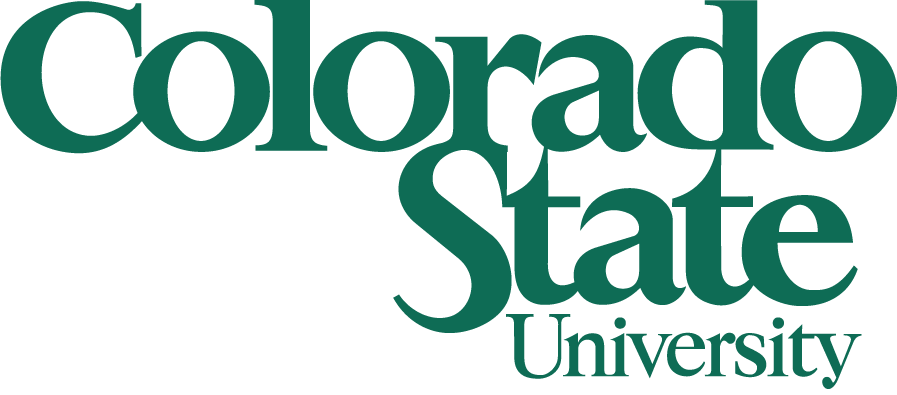# Seminar## Rocky Mountain Algebraic Combinatorics Seminar

### Weakenings of the Pasch axiom in ordered geometry

Victor Pambuccian
Arizona State University

The axiomatics of ordered geometry goes back to Moritz Pasch's first modern axiomatization of geometry of 1882. There Pasch axiomatizes plane ordered geometry by means of axioms on points and segments. His axioms, rephrased in terms of the betweenness relation, consist of axioms stipulating that lines are ordered, together with one two-dimensional order axiom, the so-called Pasch axiom (if a line intersects one of the sides of a triangle, without passing through any vertex, then it must intersect another side as well). To axiomatize higher-dimensional ordered geometries, Peano (1890), has introduced two weakenings of the Pasch axiom, which we will refer to as the inner and outer form of the Pasch axiom. This talk is concerned with the logical relationship between these axioms, as well as with Pasch's Theorem (a line cannot intersect all three sides of a triangle), with missing links between them (a missing link between an axiom A and a stronger axiom B, is an axiom C for which A and C is equivalent to B, with C satisfying some additional conditions) , and with the question regarding the simplicity of the Pasch axiom (can the Pasch axiom be replaced by an arguably simpler one? - here "simple" is defined in a syntactic manner). We will find out that, surprisingly, the Pasch axiom is not the simplest possible one. We will also encounter the non-planarity of K5 and K3,3 on our journey.

### Sum of Dilates

George Shakan
University of Wyoming

Let A be a nonempty, finite subset of the integers and q be a positive integer. Let A + q·A be all the sums a + qb such that a and b are in A. My question is: How small can |A+q.A| be? For example, it is a fun exercise to show that |A+A| is at least 2|A| − 1 and |A + 2A| is at least 3|A| −2 with equality holding in both cases for the set {1, ..., n}. I will also discuss some related problems and their connection to convex geometry, namely the Brunn-Minkowski inequality. This talk is based on joint work with Antal Balog.

Weber 223
4–6 pm
Friday, April 11, 2014
(Refreshments in Weber 117, 3:30–4 pm)

This is a joint Denver U / UC Boulder / UC Denver / U of Wyoming / CSU seminar that meets biweekly. Anyone interested is welcome to join us at a local restaurant for dinner after the talks.